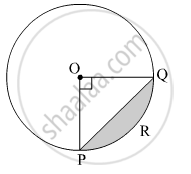Advertisement Remove all ads

# In the Given Figure, If O is the Centre of the Circle, Pq is a Chord. ∠ Poq = 90°, Area of Shaded Region is 114 Cm2 , Find the Radius of the Circle. π = 3.14) - Geometry

Advertisement Remove all ads
Advertisement Remove all ads
Advertisement Remove all ads

In the given figure, if O is the centre of the circle, PQ is a chord. $\angle$ POQ = 90°, area of shaded region is 114 cm2 , find the radius of the circle. $\pi$ = 3.14)Advertisement Remove all ads

#### Solution

∠POQ = θ = 90º
Let the radius of the circle be cm.
Area of the shaded region = Area of the segment PRQ = 114 cm2

$\therefore r^2 \left( \frac{\pi\theta}{360° } - \frac{\sin\theta}{2} \right) = 114$

$\Rightarrow r^2 \left( \frac{3 . 14 \times 90° }{360° } - \frac{\sin90° }{2} \right) = 114$

$\Rightarrow r^2 \left( \frac{3 . 14}{4} - \frac{1}{2} \right) = 114$

$\Rightarrow r^2 \times \left( 0 . 785 - 0 . 5 \right) = 114$

$\Rightarrow r = \sqrt{\frac{114}{0 . 285}}$

$\Rightarrow r = \sqrt{400} = 20 cm$

Thus, the radius of the circle is 20 cm.

Concept: Areas of Sector and Segment of a Circle
Is there an error in this question or solution?
Advertisement Remove all ads
Share
Notifications

View all notifications

Forgot password?